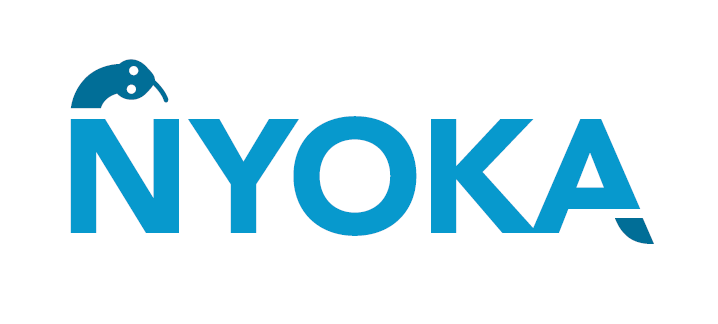# Nyoka## Overview

Nyoka is a Python library for comprehensive support of the latest PMML (PMML 4.4) standard. Using Nyoka, Data Scientists can export a large number of Machine Learning and Deep Learning models from popular Python frameworks into PMML by either using any of the numerous included ready-to-use exporters or by creating their own exporter for specialized/individual model types by simply calling a sequence of constructors.

Besides about 500 Python classes which each cover a PMML tag and all constructor parameters/attributes as defined in the standard, Nyoka also provides an increasing number of convenience classes and functions that make the Data Scientist’s life easier for example by reading or writing any PMML file in one line of code from within your favorite Python environment.

Nyoka comes to you with the complete source code in Python, extended HTML documentation for the classes/functions, and a growing number of Jupyter Notebook tutorials that help you familiarize yourself with the way Nyoka supports you in using PMML as your favorite Data Science transport file format.

Read the documentation at Nyoka Documentation.

## Prerequisites

• Python >= 3.6, < 3.8

nyoka requires:

• lxml

## Installation

You can install nyoka using:

``pip install --upgrade nyoka``

## Usage

Nyoka contains seperate exporters for each library, e.g., scikit-learn, keras, xgboost etc.

library exporter
scikit-learn _skl_topmml
xgboost _xgboost_topmml
lightgbm _lgbm_topmml
keras KerasToPmml
statsmodels StatsmodelsToPmml & ExponentialSmoothingToPmml
retinanet RetinanetToPmml

The main module of Nyoka is `nyoka`. To use it for your model, you need to import the specific exporter from nyoka as -

``from nyoka import skl_to_pmml, lgb_to_pmml #... so on``

#### Note - If scikit-learn, xgboost and lightgbm model is used then the model should be used inside sklearn's Pipeline.

The workflow is as follows (For example, a Decision Tree Classifier with StandardScaler) -

• Create scikit-learn's `Pipeline` object and populate it with any pre-processing steps and the model object.

``````from sklearn.pipeline import Pipeline
from sklearn.tree import DecisionTreeClassifier
from sklearn.preprocessing import StandardScaler
pipeline_obj = Pipeline([
("scaler",StandardScaler()),
("model",DecisionTreeClassifier())
])``````
• Call `Pipeline.fit(X,y)` method to train the model.

``````from sklearn.dataset import load_iris
X = iris_data.data
y = iris_data.target
features = iris_data.feature_names
pipeline_obj.fit(X,y)``````
• Use the specific exporter and pass the pipeline object, feature names of the training dataset, target name and expected name of the PMML to the exporter function. If target name is not given default value `target` is used. Similarly, for pmml name, default value `from_sklearn.pmml`/`from_xgboost.pmml`/`from_lighgbm.pmml` is used.

``````from nyoka import skl_to_pmml
skl_to_pmml(pipeline=pipeline_obj,col_names=features,target_name="species",pmml_f_name="decision_tree.pmml")``````

#### For Keras, RetinaNet and Statsmodels, pipeline is not required. The fitted model needs to be passed to the exporter.

``````import pandas as pd
from statsmodels.tsa.arima_model import ARIMA
from nyoka import StatsmodelsToPmml
sales_data = pd.read_csv('sales-cars.csv', index_col=0, parse_dates = True)
model = ARIMA(sales_data, order = (4, 1, 2))
result = model.fit()
StatsmodelsToPmml(result,"Sales_cars_ARIMA.pmml")``````

## Examples

Example jupyter notebooks can be found in `nyoka/examples`. These files contain code to showcase how to use different exporters.

• Exporting `scikit-learn` models into PMML

• Exporting `Keras` models into PMML

• Exporting `XGBoost` model into PMML

• Exporting `LightGBM` model into PMML

• Exporting `statsmodels` model into PMML

• Exporting `retinanet` model into PMML

## Nyoka Submodules

Nyoka contains one submodule called `preprocessing`. This module contains preprocessing classes implemented by Nyoka. Currently there is only one preprocessing class, which is `Lag`.

#### What is Lag? When to use it?

Lag is a preprocessing class implemented by Nyoka. When used inside scikit-learn's pipeline, it simply applies an `aggregation` function for the given features of the dataset by combining `value` number of previous records. It takes two arguments- aggregation and value.

The valid `aggregation` functions are - "min", "max", "sum", "avg", "median", "product" and "stddev".

To use Lag -

• Import it from nyoka -
``from nyoka.preprocessing import Lag``
• Create an instance of Lag -
``````lag_obj = Lag(aggregation="sum", value=5)
'''
This means taking previous 5 values and perform `sum`. When used inside pipeline, this will be applied to all the columns.
If used inside DataFrameMapper, the it will be applied to only those columns which are inside DataFrameMapper.
'''``````
• Use this object inside scikit-learn's pipeline to train.
``````from sklearn.pipeline import Pipeline
from sklearn.tree import DecisionTreeClassifier
from nyoka.preprocessing import Lag
pipeline_obj = Pipeline([
("lag",Lag(aggregation="sum",value=5)),
("model",DecisionTreeClassifier())
])``````

## Uninstallation

``pip uninstall nyoka``